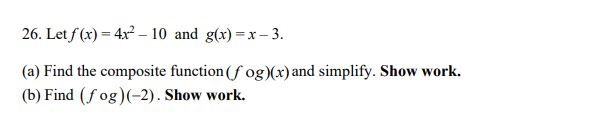# 26. Let f (x) = 4x – 10 and g(x) =x– 3.(a) Find the composite function (f og)(x) and simplify. Show work.(b) Find (f og)(-2). Show work.

Question
14 views

practice question 26help_outlineImage Transcriptionclose26. Let f (x) = 4x – 10 and g(x) =x– 3. (a) Find the composite function (f og)(x) and simplify. Show work. (b) Find (f og)(-2). Show work. fullscreen
check_circle

Step 1

The given functions are,

Step 2

(a) The required composite functi...

### Want to see the full answer?

See Solution

#### Want to see this answer and more?

Solutions are written by subject experts who are available 24/7. Questions are typically answered within 1 hour.*

See Solution
*Response times may vary by subject and question.
Tagged in

### Equations and In-equations